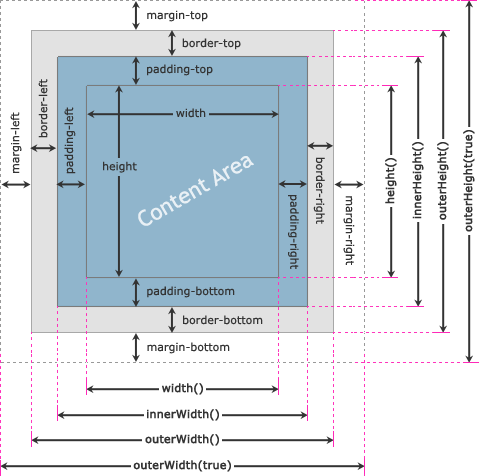JQUERY BASIC
JQUERY EFFECTS
JQUERY MANIPULATION
JQUERY EXAMPLES
JQUERY REFERENCE

# jQuery Dimensions

In this tutorial you will learn how to get or set dimensions of an element's box such as width and height using jQuery.

## Understanding the jQuery Dimensions

jQuery provides several methods, such as `height()`, `innerHeight()`, `outerHeight()`, `width()`, `innerWidth()` and `outerWidth()` to get and set the CSS dimensions for the elements. Check out the following illustration to understand how these methods are calculating the dimensions of an element's box.## jQuery `width()` and `height()` Methods

The jQuery `width()` and `height()` methods get or set the `width` and the `height` of the element respectively. This width and height doesn't include `padding`, `border` and `margin` on the element. The following example will return the width and height of a `<div>` element.

#### Example

Try this code »
``````<script>
\$("button").click(function(){
var divWidth = \$("#box").width();
var divHeight = \$("#box").height();
\$("#result").html("Width: " + divWidth + ", " + "Height: " + divHeight);
});
});
</script>``````

Similarly, you can set the width and height of the element by including the value as a parameter within the `width()` and `height()` method. The value can be either a string (number and unit e.g. 100px, 20em, etc.) or a number. The following example will set the width of a `<div>` element to 400 pixels and height to 300 pixels respectively.

#### Example

Try this code »
``````<script>
\$("button").click(function(){
\$("#box").width(400).height(300);
});
});
</script>``````

Note: Use the jQuery `width()` or `height()` method if you want to use an element's width or height in a mathematical calculation, since it returns the `width` and `height` property value as a unit-less pixel value (e.g. 400). Whereas, the `css("width")` or `css("height")` methods returns value with units (e.g. 400px).

## jQuery `innerWidth()` and `innerHeight()` Methods

The jQuery `innerWidth()` and `innerHeight()` methods get or set the inner width and the inner height of the element respectively. This inner width and height includes the `padding` but excludes `border` and `margin` on the element. The following example will return the inner width and height of a `<div>` element on the click of a button.

#### Example

Try this code »
``````<script>
\$("button").click(function(){
var divWidth = \$("#box").innerWidth();
var divHeight = \$("#box").innerHeight();
\$("#result").html("Inner Width: " + divWidth + ", " + "Inner Height: " + divHeight);
});
});
</script>``````

Similarly, you can set the element's inner width and height by passing the value as a parameter to the `innerWidth()` and `innerHeight()` method. These methods only alter the width or height of the element's content area to match the specified value.

For example, if the current width of the element is 300 pixels and the sum of the left and right padding is equal to 50 pixels than the new width of the element after setting the inner width to 400 pixels is 350 pixels i.e. `New Width = Inner Width - Horizontal Padding`. Similarly, you can estimate the change in height while setting the inner height.

#### Example

Try this code »
``````<script>
\$("button").click(function(){
\$("#box").innerWidth(400).innerHeight(300);
});
});
</script>``````

## jQuery `outerWidth()` and `outerHeight()` Methods

The jQuery `outerWidth()` and `outerHeight()` methods get or set the outer width and the outer height of the element respectively. This outer width and height includes `padding` and `border` but excludes the `margin` on the element. The following example will return the outer width and height of a `<div>` element on the click of a button.

#### Example

Try this code »
``````<script>
\$("button").click(function(){
var divWidth = \$("#box").outerWidth();
var divHeight = \$("#box").outerHeight();
\$("#result").html("Outer Width: " + divWidth + ", " + "Outer Height: " + divHeight);
});
});
</script>``````

You can also get the outer width and height that includes `padding` and `border` as well as the `margin` of the element. For that just specify the `true` parameter for the outer width methods, like `outerWidth(true)` and `outerHeight(true)`.

#### Example

Try this code »
``````<script>
\$("button").click(function(){
var divWidth = \$("#box").outerWidth(true);
var divHeight = \$("#box").outerHeight(true);
\$("#result").html("Outer Width: " + divWidth + ", " + "Outer Height: " + divHeight);
});
});
</script>``````

Similarly, you can set the element's outer width and height by passing the value as a parameter to the `outerWidth()` and `outerHeight()` methods. These methods only alter the width or height of the element's content area to match the specified value, like the `innerWidth()` and `innerHeight()` methods.

For example, if the current width of the element is 300 pixels, and the sum of the left and right padding is equal to 50 pixels, and the sum of the width of the left and right border is 20 pixels than the new width of the element after setting the outer width to 400 pixels is 330 pixels i.e. `New Width = Outer Width - (Horizontal Padding + Horizontal Border)`. Similarly, you can estimate the change in height while setting the outer height.

#### Example

Try this code »
``````<script>